Cube A cube is a solid with six square sides. [Figure 1-25] A cube is just a special type of rectangular solid. It has the same formula for volume as does the rectangular solid which is Volume = Length × Width × Height = L × W × H. Because all of the sides of a cube are equal, the volume formula for a cube can also be written as: Volume = Side × Side × Side = S3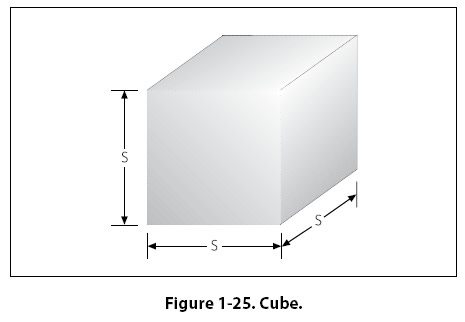Example: A large, cube-shaped carton contains a shipment of smaller boxes inside of it. Each of the smaller boxes is 1 ft × 1 ft × 1 ft. The measurement of the large carton is 3 ft × 3 ft × 3 ft. How many of the smaller boxes are in the large carton? First, substitute the known values into the formula.   V = L × W × H      = 3 ft × 3 ft × 3 ft      = 27 cubic feet of volume in the large carton Since each of the smaller boxes has a volume of 1 cubic foot, the large carton will hold 27 boxes. Cylinder A solid having the shape of a can, or a length of pipe, or a barrel is called a cylinder. [Figure 1-26] The ends of a cylinder are identical circles. The formula for the volume of a cylinder is: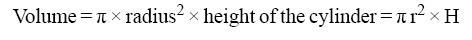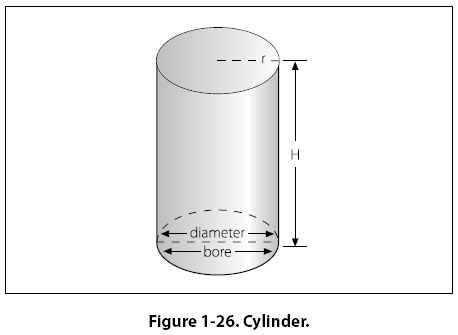One of the most important applications of the volume of a cylinder is finding the piston displacement of a cylinder in a reciprocating engine. Piston displacement is the total volume (in cubic inches, cubic centimeters, or liters) swept by all of the pistons of a reciprocating engine as they move in one revolution of the crankshaft. The formula for piston displacement is given as: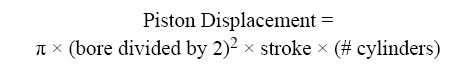The bore of an engine is the inside diameter of the cylinder. The stroke of the engine is the length the piston travels inside the cylinder. [Figure 1-27]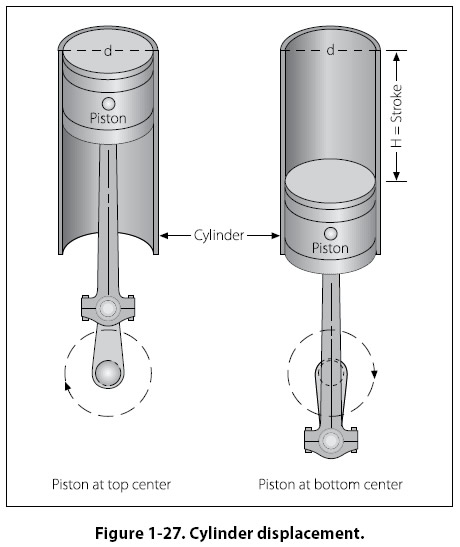Example: Find the piston displacement of one cylinder in a multi-cylinder aircraft engine. The engine has a cylinder bore of 5.5 inches and a stroke of 5.4 inches. First, substitute the known values in the formula.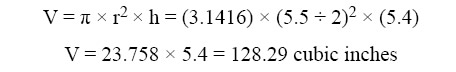The piston displacement of one cylinder is 128.29 cubic inches. For an eight cylinder engine, then the total engine displacement would be: Total Displacement for 8 cylinders = 8 × 128.29 = 1026.32 cubic inches of displacement
 ©AvStop Online Magazine                                                                                                                                                      Contact Us              Return To Books